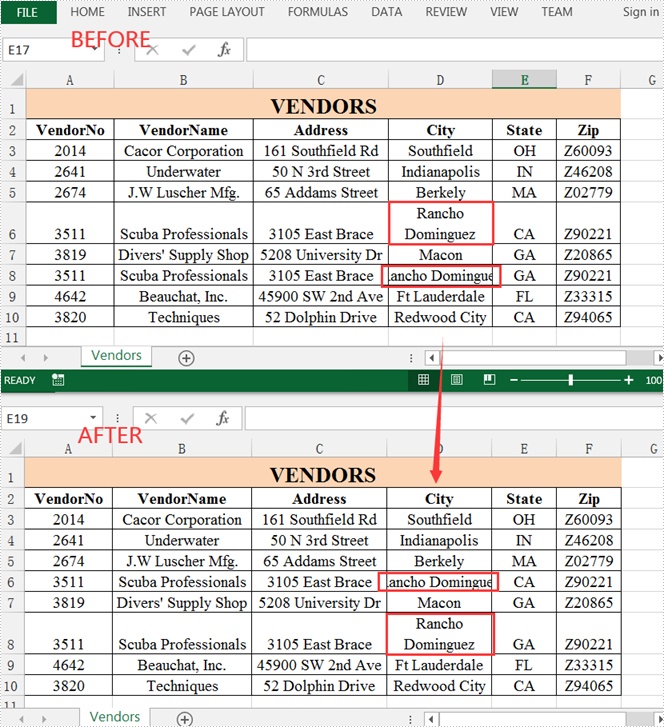Tuesday, 28 September 2021 02:49

Java: Accept or Reject Tracked Changes in Excel

An Excel document with Track Changes turned on will let you know what changes have been made to the document since the author has saved it. If you have the full authority over the document, you can accept or reject each revision. This article covers how to accept or reject all tracked changes at once using Spire.XLS for Java.

Install Spire.XLS for Java

First of all, you're required to add the Spire.Xls.jar file as a dependency in your Java program. The JAR file can be downloaded from this link. If you use Maven, you can easily import the JAR file in your application by adding the following code to your project's pom.xml file.

• Package Manager
<repositories>
<repository>
<id>com.e-iceblue</id>
<name>e-iceblue</name>
<url>http://repo.e-iceblue.com/nexus/content/groups/public/</url>
</repository>
</repositories>
<dependencies>
<dependency>
<groupId>e-iceblue</groupId>
<artifactId>spire.xls</artifactId>
<version>4.9.0</version>
</dependency>
</dependencies>

Accept Tracked Changes in a Workbook

To determine whether a workbook has tracked changes, use Workbook.hasTrackedChanegs() method. If yes, you can accept all changes at once using Workbook.acceptAllTrackedchanges() method. The following are the steps to accept tracked changes in an Excel workbook.

• Create a Workbook object.
• Determine if the workbook has tracked changes by Workbook.hasTrackedChanegs() method.
• Accept tracked changes using Workbook.acceptAllTrackedChanges() method.
• Save the document to another file using Workbook.saveToFile() method.
• Java
import com.spire.xls.FileFormat;
import com.spire.xls.Workbook;

public class AcceptTrackedChanges {

public static void main(String[] args) {

//Create a Workbook object
Workbook wb = new Workbook();

//Determine if the workbook has tracked changes
if (wb.hasTrackedChanges())
{
//Accept tracked changes in the workbook
wb.acceptAllTrackedChanges();
}

//Save to file
wb.saveToFile("output/AcceptChanges.xlsx", FileFormat.Version2013);
}
}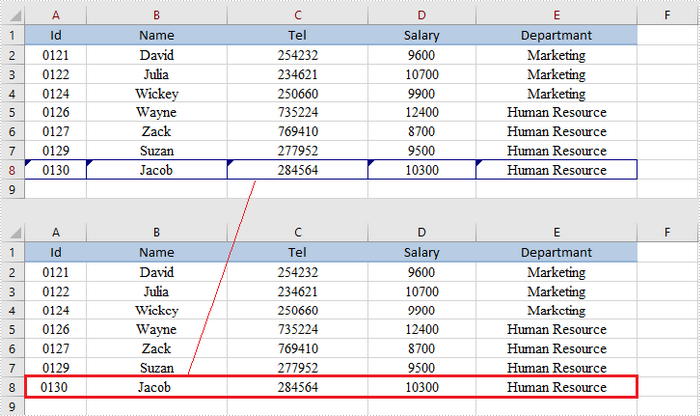Reject Tracked Changes in a Workbook

If the tracked changes have been proven to exist in a workbook, you can reject them using Workbook.rejectAllTrackedChanges() method. The following are the steps to achieve this.

• Create a Workbook object.
• Determine if the workbook has tracked changes by Workbook.hasTrackedChanegs() method.
• Reject all tracked changes using Workbook.rejectAllTrackedChanges() method.
• Save the document to another file using Workbook.saveToFile() method.
• Java
import com.spire.xls.FileFormat;
import com.spire.xls.Workbook;

public class RejectTrackedChanges {

public static void main(String[] args) {

//Create a Workbook object
Workbook wb = new Workbook();

//Determine if the workbook has tracked changes
if (wb.hasTrackedChanges())
{
//Reject tracked changes in the workbook
wb.rejectAllTrackedChanges();
}

//Save to file
wb.saveToFile("output/RejectChanges.xlsx", FileFormat.Version2013);
}
}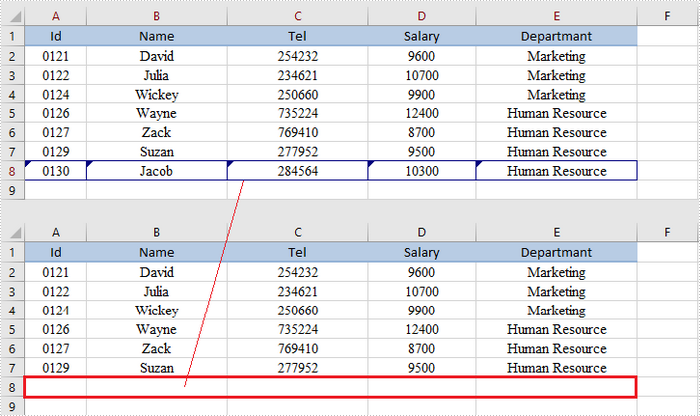If you'd like to remove the evaluation message from the generated documents, or to get rid of the function limitations, please request a 30-day trial license for yourself.

Wednesday, 22 September 2021 06:39

C#/VB.NET: Add Math Equations to PowerPoint using LaTeX Code

LaTeX is a powerful tool to typeset mathematical equations. It supports plenty of mathematical symbols and notations to create mathematical equations, for instance, fractions, integrals and more.

Spire.Presentation API provides developers with the ability to create and add mathematical equations to PowerPoint shape using LaTeX code. The following steps demonstrate how to achieve this function using Spire.Presentation:

• Create a Presentation instance.
• Get the reference of a slide by using its index.
• Use ShapeList.AppendShape method to add a shape to the first slide.
• Use ParagraphCollection.AddParagraphFromLatexMathCode(string) method to create a mathematical equation from LaTeX code and add it to the shape.
• Save the result document using Presentation.SaveToFile(string, FileFormat) method.

The following code shows how to add mathematical equations to PowerPoint in C#.

using Spire.Presentation;
using System.Drawing;

namespace MathEquations
{
class Program
{
static void Main(string[] args)
{
//The LaTeX codes
string latexCode1 = @"x^{2} + \sqrt{x^{2}+1}=2";
string latexCode2 = @"F(x) &= \int^a_b \frac{1}{3}x^3";
string latexCode3 = @"\alpha + \beta  \geq \gamma";
string latexCode4 = @"\overrightarrow{abc}";
string latexCode5 = @"\begin{bmatrix} 1 & 0 & \cdots & 0\\ 1 & 0 & \cdots & 0\\ \vdots & \vdots & \ddots & \vdots\\ 1 & 0 & 0 & 0 \end{bmatrix}";
string latexCode6 = @"\log_a{b}";

//Create a Presentation instance
Presentation ppt = new Presentation();

//Get the first slide by using its index
ISlide slide = ppt.Slides;

//Add a shape to the slide
IAutoShape shape = slide.Shapes.AppendShape(ShapeType.Rectangle, new RectangleF(30, 100, 200, 30));
shape.TextFrame.Paragraphs.Clear();
//Add a math equation to the shape using the LaTeX code

//Add a shape to the slide
shape = slide.Shapes.AppendShape(ShapeType.Rectangle, new RectangleF(240, 100, 200, 40));
shape.TextFrame.Paragraphs.Clear();
//Add a math equation to the shape using the LaTeX code

//Add a shape to the slide
shape = slide.Shapes.AppendShape(ShapeType.Rectangle, new RectangleF(30, 180, 200, 40));
shape.TextFrame.Paragraphs.Clear();
//Add a math equation to the shape using the LaTeX code

//Add a shape to the slide
shape = slide.Shapes.AppendShape(ShapeType.Rectangle, new RectangleF(240, 180, 200, 40));
shape.TextFrame.Paragraphs.Clear();
//Add a math equation to the shape using the LaTeX code

//Add a shape to the slide
shape = slide.Shapes.AppendShape(ShapeType.Rectangle, new RectangleF(30, 280, 200, 70));
shape.TextFrame.Paragraphs.Clear();
//Add a math equation to the shape using the LaTeX code

//Add a shape to the slide
shape = slide.Shapes.AppendShape(ShapeType.Rectangle, new RectangleF(240, 280, 200, 40));
shape.TextFrame.Paragraphs.Clear();
//Add a math equation to the shape using the LaTeX code

for (int i = 0; i < slide.Shapes.Count; i++)
{
slide.Shapes[i].Fill.FillType = Spire.Presentation.Drawing.FillFormatType.None;
slide.Shapes[i].Line.FillType = Spire.Presentation.Drawing.FillFormatType.None;
}

//Save the result document
ppt.SaveToFile("MathEquations.pptx", FileFormat.Pptx2013);
}
}
}

The following code shows how to add mathematical equations to PowerPoint in VB.NET.

Imports Spire.Presentation
Imports System.Drawing

Namespace MathEquations
Friend Class Program
Private Shared Sub Main(ByVal args As String())
'The LaTeX codes
Dim latexCode1 As String = "x^{2} + \sqrt{x^{2}+1}=2"
Dim latexCode2 As String = "F(x) &= \int^a_b \frac{1}{3}x^3"
Dim latexCode3 As String = "\alpha + \beta  \geq \gamma"
Dim latexCode4 As String = "\overrightarrow{abc}"
Dim latexCode5 As String = "\begin{bmatrix} 1 & 0 & \cdots & 0\\ 1 & 0 & \cdots & 0\\ \vdots & \vdots & \ddots & \vdots\\ 1 & 0 & 0 & 0 \end{bmatrix}"
Dim latexCode6 As String = "\log_a{b}"

'Create a Presentation instance
Dim ppt As Presentation = New Presentation()

'Get the first slide by using its index
Dim slide As ISlide = ppt.Slides(0)

'Add a shape to the slide
Dim shape As IAutoShape = slide.Shapes.AppendShape(ShapeType.Rectangle, New RectangleF(30, 100, 200, 30))
shape.TextFrame.Paragraphs.Clear()
'Add a math equation to the shape using the LaTeX code

'Add a shape to the slide
shape = slide.Shapes.AppendShape(ShapeType.Rectangle, New RectangleF(240, 100, 200, 40))
shape.TextFrame.Paragraphs.Clear()
'Add a math equation to the shape using the LaTeX code

'Add a shape to the slide
shape = slide.Shapes.AppendShape(ShapeType.Rectangle, New RectangleF(30, 180, 200, 40))
shape.TextFrame.Paragraphs.Clear()
'Add a math equation to the shape using the LaTeX code

'Add a shape to the slide
shape = slide.Shapes.AppendShape(ShapeType.Rectangle, New RectangleF(240, 180, 200, 40))
shape.TextFrame.Paragraphs.Clear()
'Add a math equation to the shape using the LaTeX code

'Add a shape to the slide
shape = slide.Shapes.AppendShape(ShapeType.Rectangle, New RectangleF(30, 280, 200, 70))
shape.TextFrame.Paragraphs.Clear()
'Add a math equation to the shape using the LaTeX code

'Add a shape to the slide
shape = slide.Shapes.AppendShape(ShapeType.Rectangle, New RectangleF(240, 280, 200, 40))
shape.TextFrame.Paragraphs.Clear()
'Add a math equation to the shape using the LaTeX code

For i As Integer = 0 To slide.Shapes.Count - 1
slide.Shapes(i).Fill.FillType = Spire.Presentation.Drawing.FillFormatType.None
slide.Shapes(i).Line.FillType = Spire.Presentation.Drawing.FillFormatType.None
Next

'Save the result document
ppt.SaveToFile("MathEquations.pptx", FileFormat.Pptx2013)
End Sub
End Class
End Namespace

The following is the output document after adding mathematical equations: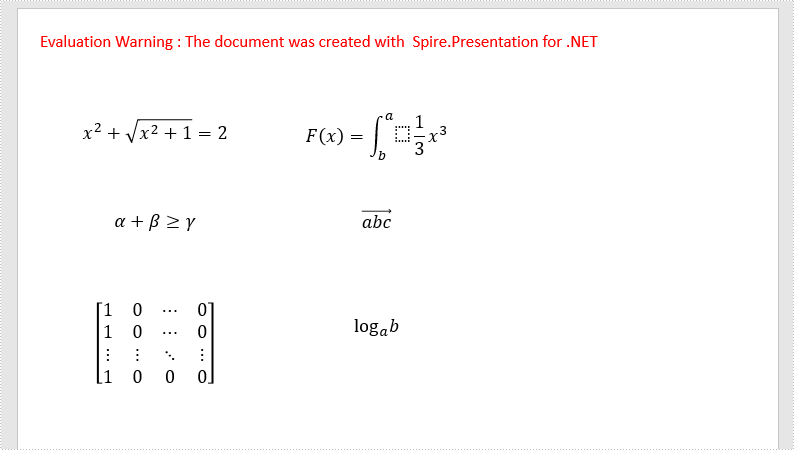If you'd like to remove the evaluation message from the generated documents, or to get rid of the function limitations, please request a 30-day trial license for yourself.

Monday, 06 September 2021 09:01

Detect and Remove Blank Pages in PDF in Java

Spire.PDF for Java provides a PdfPageBase.isBlank method to detect if a PDF page is absolutely blank, however, some pages look like “blank” but they actually contain white images, these pages won't be deemed as blank using the PdfPageBase.isBlank method. Therefore, we create a custom method isBlankImage to use it in conjunction with the PdfPageBase.isBlank method to detect blank and those white but non-blank pages.

In the following example, we will describe how to detect and remove blank and white pages in a PDF document using Spire.PDF for Java.

Note: This solution will convert PDF pages into images and detect if an image is blank. It is necessary to apply a license to remove the evaluation message in the converted images. Otherwise, this method won't work properly. If you do not have a license, contact sales@e-iceblue.com for a temporary one for evaluation purpose.

import com.spire.pdf.PdfDocument;
import com.spire.pdf.PdfPageBase;
import com.spire.pdf.graphics.PdfImageType;

import java.awt.*;
import java.awt.image.BufferedImage;

public class RemoveBlankPages {
public static void main(String []args){
//Create a PdfDocument instance
PdfDocument pdf = new PdfDocument();

BufferedImage image;
//Iterate through pages in the PDF
for(int i = pdf.getPages().getCount()-1; i>=0; i--)
{
PdfPageBase page = pdf.getPages().get(i);
//Detect if a page is blank
if(page.isBlank())
{
//If yes, remove the page
pdf.getPages().remove(page);
}
else
{
//Save PDF page as image
image = pdf.saveAsImage(i, PdfImageType.Bitmap);

//Detect if a page contains white image
if (isBlankImage(image))
{
//If yes, remove the page
pdf.getPages().remove(page);
}
}

}
//Save the result document
pdf.saveToFile("RemoveBlankPages.pdf");
}
//Detect if an image is blank
public static boolean isBlankImage(BufferedImage image)
{
BufferedImage bufferedImage = image;

Color pixel;
for (int i = 0; i < bufferedImage.getWidth(); i++)
{
for (int j = 0; j < bufferedImage.getHeight(); j++)
{
pixel = new Color(bufferedImage.getRGB(i, j));
if (pixel.getRed() < 240 || pixel.getGreen() < 240 || pixel.getBlue() < 240)
{
return false;
}
}
}
return true;
}
}

The input PDF: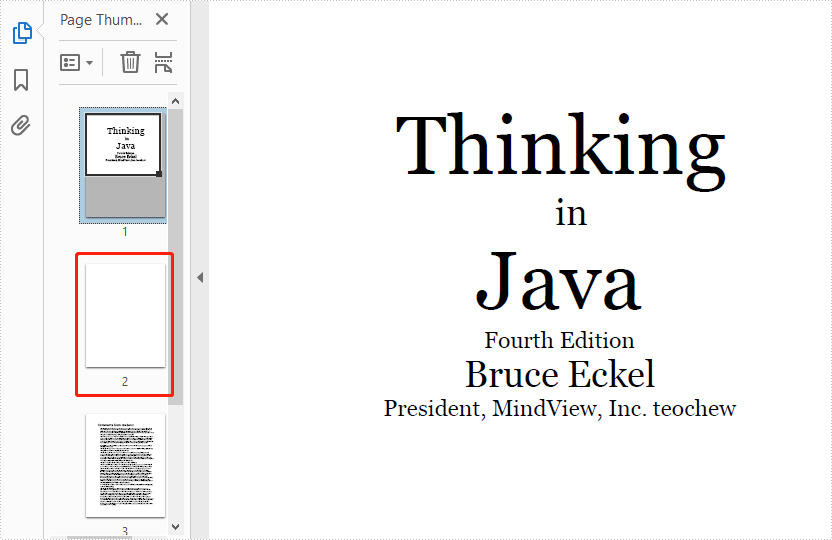The output PDF: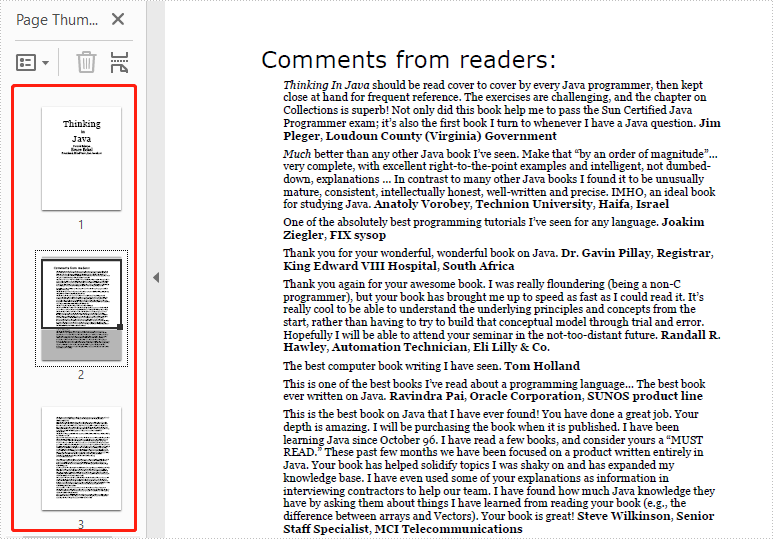Monday, 06 September 2021 08:50

Set the Slide Size in PowerPoint in Java

SlideSize.setType() method is used to set or change the slide size to a predefined size like Widescreen 16x9, Overhead, A3, A4, Banner, B4, and B5. To customize the slide size, set the size type to custom, and then apply a customized width and height to slide using the SlideSize.setSize() method.

Set slide size to a predefined size

import com.spire.presentation.*;

import java.awt.geom.Rectangle2D;

public class SetToPredefinedSize {
public static void main(String[] args) throws Exception {

//Create a Presentation object
Presentation presentation = new Presentation();

//Set the slide size to Screen 16x9
presentation.getSlideSize().setType(SlideSizeType.SCREEN_16_X_9);

//Add a shape to the first slide
IAutoShape shape = presentation.getSlides().get(0).getShapes().appendShape(ShapeType.RECTANGLE, new Rectangle2D.Float(20, 50, 500, 50));
shape.getTextFrame().setText("This example shows you how to set slide size to Widescreen 16X9.");

//Save to file
presentation.saveToFile("output/PredefinedSize.pptx", FileFormat.PPTX_2013);
}
}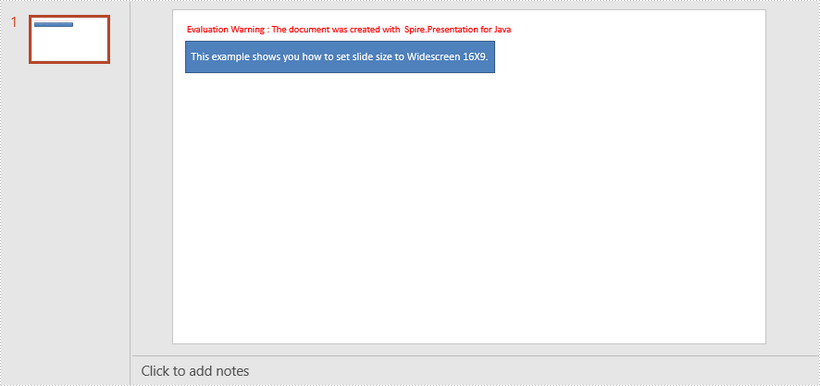Customize slide size

import com.spire.presentation.*;

import java.awt.*;
import java.awt.geom.Rectangle2D;

public class CustomizeSlideSize {

public static void main(String[] args) throws Exception {

//Create a Presentation object
Presentation presentation = new Presentation();

//Set the slide size type to custom
presentation.getSlideSize().setType(SlideSizeType.CUSTOM);

//Set the slide size to a custom size
presentation.getSlideSize().setSize(new Dimension(800,400));

//Add a shape to the first slide
IAutoShape shape = presentation.getSlides().get(0).getShapes().appendShape(ShapeType.RECTANGLE, new Rectangle2D.Float(20, 50, 500, 50));
shape.getTextFrame().setText("This example shows you how to customize the slide size.");

//Save to file
presentation.saveToFile("output/CustomSize.pptx", FileFormat.PPTX_2013);
}
}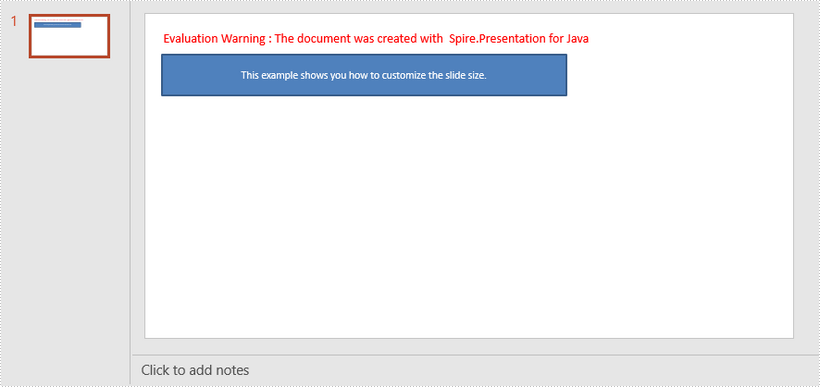Monday, 30 August 2021 09:47

Copy Formatting from One Cell Range to Another in Java

Spire.XLS for Java provides the getStyle() method and setStyle() method under the IXLSRange interface to get or set the style of a specific cell range. To copy formatting from one cell to another, get the style first and then apply it to another cell.

import com.spire.xls.ExcelVersion;
import com.spire.xls.Workbook;
import com.spire.xls.Worksheet;

public class CopyCellFormatting {
public static void main(String[] args) {

//Create a Workbook object
Workbook workbook = new Workbook();

//Get the first worksheet
Worksheet sheet = workbook.getWorksheets().get(0);

//Get the number of rows used
int rowCount = sheet.getRows().length;

//Loop through the rows
for (int i = 1; i < rowCount + 1; i++)
{
//Copy the formatting from a certain cell to another
sheet.getRange().get(String.format("C%d",i)).setStyle(sheet.getRange().get(String.format("A%d",i)).getStyle());
}

//Save the result to file
workbook.saveToFile("output/CopyFormatting.xlsx", ExcelVersion.Version2016);
}
}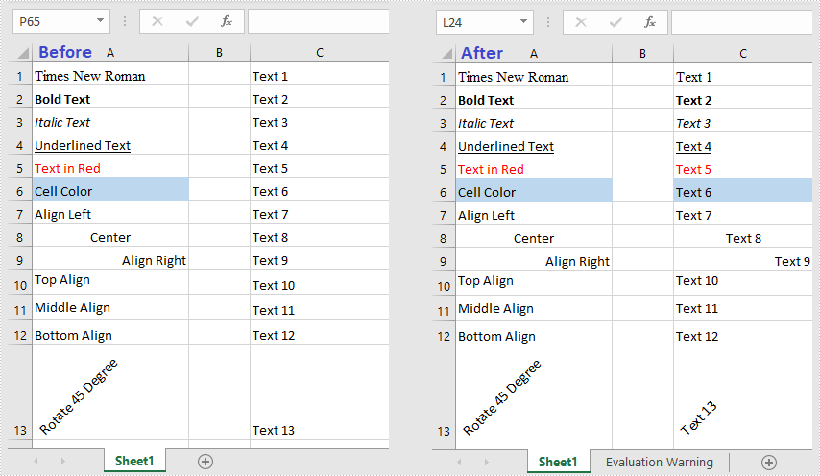Monday, 30 August 2021 06:22

Shrink Text to Fit in a Cell in Excel in Java

Spire.XLS for Java provides you with the ability to shrink text to fit in a cell by using the setShrinkToFit method of the CellStyleObject class. The setShrinkToFit method accepts the following parameter:

boolean: specify whether to shrink text to fit in a cell.

The following example shows how to shrink text to fit in a cell in Excel using Spire.XLS for Java.

import com.spire.xls.*;

public class ShrinkTextToFitInACell {
public static void main(String []args) throws Exception {
//Create a workbook instance
Workbook workbook = new Workbook();

//Get the first worksheet
Worksheet sheet = workbook.getWorksheets().get(0);

//Get the cell range to shrink text
CellRange cell = sheet.getRange().get("B2:B3");

//Enable “shrink to fit”
cell.getCellStyle().setShrinkToFit(true);

//Save the file
workbook.saveToFile("ShrinkTextToFitInACell.xlsx", ExcelVersion.Version2013);
}
}

The input Excel: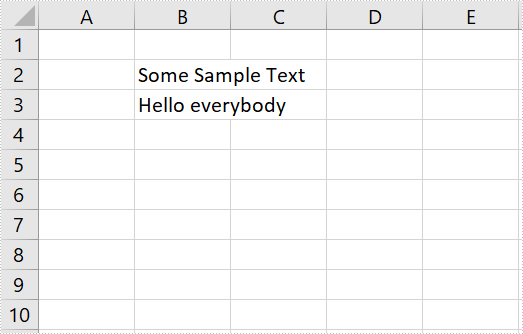The output Excel: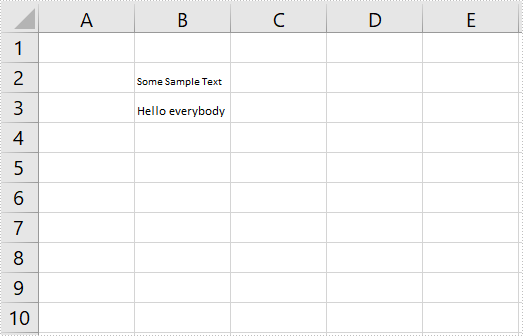Tuesday, 24 August 2021 06:51

Change Font Styles in PowerPoint in Java

This article demonstrates how to change font styles (font name, font size, font color, bold, italic and underlined) of an existing PowerPoint document by using Spire.Presentation for Java.

import com.spire.presentation.*;
import com.spire.presentation.drawing.FillFormatType;

import java.awt.*;

public class ChangeFontStyles {

public static void main(String[] args) throws Exception {

//Create a Presentation object
Presentation presentation = new Presentation();

//Get the text shape
IAutoShape shape = (IAutoShape) presentation.getSlides().get(0).getShapes().get(0);

//Get the first paragraph and change the font color of it
ParagraphEx paragraph = shape.getTextFrame().getParagraphs().get(0);
PortionEx textRange =  paragraph.getFirstTextRange();
textRange.getFormat().getFill().setFillType(FillFormatType.SOLID);
textRange.getFormat().getFill().getSolidColor().setColor(Color.blue);

//Get the second paragraph and make the text bold, italic and unlined
paragraph = shape.getTextFrame().getParagraphs().get(1);
textRange = paragraph.getFirstTextRange();
textRange.getFormat().isBold(TriState.TRUE);
textRange.getFormat().isItalic(TriState.TRUE);
textRange.getFormat().setTextUnderlineType(TextUnderlineType.DASHED);

//Get the third paragraph and change the font name and size
paragraph = shape.getTextFrame().getParagraphs().get(2);
textRange = paragraph.getFirstTextRange();
textRange.getFormat().setLatinFont(new TextFont("Segoe Print"));
textRange.getFormat().setFontHeight(22f);

//Save the document
presentation.saveToFile("output/ChangeFontStyles.pptx", FileFormat.PPTX_2013);
}
}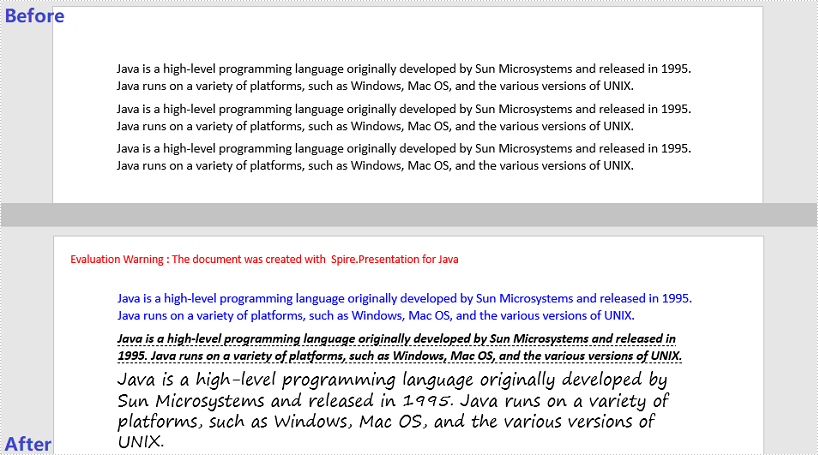Monday, 23 August 2021 07:49

Replace Text with Regular Expression (Regex) in PowerPoint in C#, VB.NET

Spire.Presentation for .NET provides you with the ability to replace text with regular expression using the ReplaceTextWithRegex method of IShape class. The ReplaceTextWithRegex method accepts the following parameters:

Regex: the regular expression to search text.

string: the text to replace with.

The following example demonstrates how to replace text with regular expression in a PowerPoint document using Spire.Presentation for .NET.

C#
using Spire.Presentation;
using System.Text.RegularExpressions;

namespace ReplaceTextWithRegex
{
class Program
{
static void Main(string[] args)
{
//Create a Presentation instance
Presentation ppt = new Presentation();

//Get the first slide
ISlide slide = ppt.Slides;

//Replace "ABC" and the subsequent to the end of the line as "ABC DEF"
Regex regex = new Regex("ABC.*");
string newvalue = "ABC DEF";
foreach (IShape shape in slide.Shapes)
{
shape.ReplaceTextWithRegex(regex, newvalue);
}

//Save the result document
ppt.SaveToFile("ReplaceTextWithRegex.pptx", FileFormat.Pptx2013);
}
}
}
VB.NET
Imports Spire.Presentation
Imports System.Text.RegularExpressions

Namespace ReplaceTextWithRegex
Friend Class Program
Private Shared Sub Main(ByVal args As String())
'Create a Presentation instance
Dim ppt As Presentation = New Presentation()

'Get the first slide
Dim slide As ISlide = ppt.Slides(0)

'Replace "ABC" and the subsequent to the end of the line as "ABC DEF"
Dim regex As Regex = New Regex("ABC.*")
Dim newvalue As String = "ABC DEF"

For Each shape As IShape In slide.Shapes
shape.ReplaceTextWithRegex(regex, newvalue)
Next

'Save the result document
ppt.SaveToFile("ReplaceTextWithRegex.pptx", FileFormat.Pptx2013)
End Sub
End Class
End Namespace

The input PowerPoint document: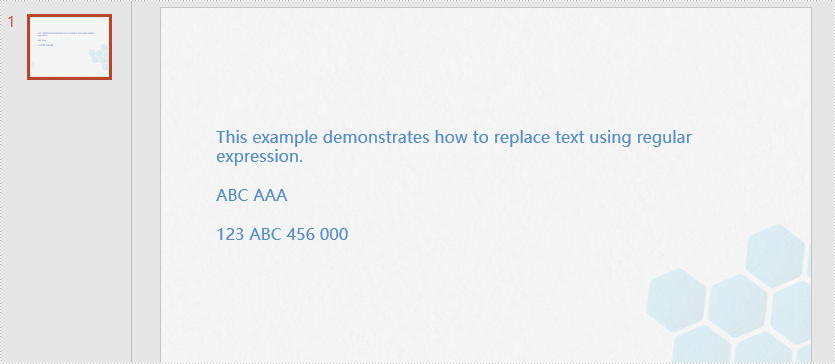The output PowerPoint document: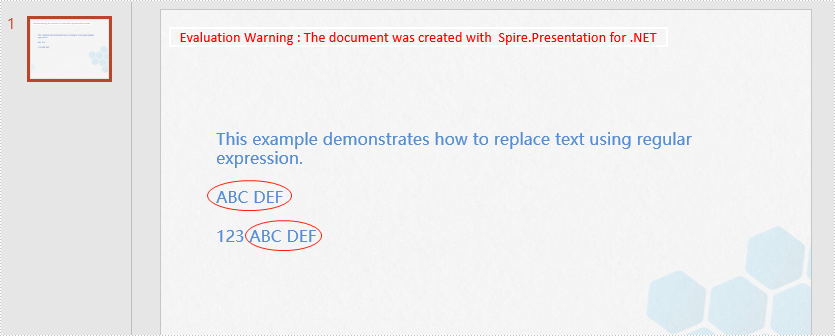Wednesday, 18 August 2021 06:16

Add Multiline Watermarks to PowerPoint in Java

This article demonstrates how to add multiline watermarks to a PowerPoint slide using Spire.Presentation for Java. To add watermarks to all slides, use one more for loop outside the two for loops in the following code snippet.

import com.spire.pdf.graphics.PdfTrueTypeFont;
import com.spire.presentation.*;
import com.spire.presentation.drawing.FillFormatType;

import java.awt.*;
import java.awt.geom.Dimension2D;
import java.awt.geom.Rectangle2D;

public static void main(String[] args) throws Exception {

//Create a Presentation object
Presentation presentation = new Presentation();

//Specify watermark text
String watermarkText = "E-iceblue";

//Get the size of the watermark text
Font font = new java.awt.Font("Arial", java.awt.Font.BOLD, 20);
PdfTrueTypeFont trueTypeFont = new PdfTrueTypeFont(font);
Dimension2D strSize = trueTypeFont.measureString(watermarkText);

//Initialize x and y coordinate
float x = 30;
float y = 80;

for (int rowNum = 0; rowNum < 4; rowNum++) {
for (int colNum = 0; colNum < 5; colNum++) {

Rectangle2D rect = new Rectangle2D.Float(x, y, (float) strSize.getWidth() + 10, (float) strSize.getHeight());
IAutoShape shape = presentation.getSlides().get(0).getShapes().appendShape(ShapeType.RECTANGLE, rect);

//Set the style of the shape
shape.getFill().setFillType(FillFormatType.NONE);
shape.getShapeStyle().getLineColor().setColor(new Color(1, 1, 1, 0));
shape.setRotation(-45);
shape.getLocking().setSelectionProtection(true);
shape.getLine().setFillType(FillFormatType.NONE);

//Add watermark text to the shape
shape.getTextFrame().setText(watermarkText);
PortionEx textRange = shape.getTextFrame().getTextRange();

//Set the style of the text range
textRange.getFill().setFillType(FillFormatType.SOLID);
textRange.getFill().getSolidColor().setColor(Color.pink);
textRange.setLatinFont(new TextFont(trueTypeFont.getName()));
textRange.setFontMinSize(trueTypeFont.getSize());

x += (100 + strSize.getWidth());

}
x = 30;
y += (100 + strSize.getHeight());
}

//Save the document
presentation.saveToFile("output/Watermark.pptx", FileFormat.PPTX_2013);
}
}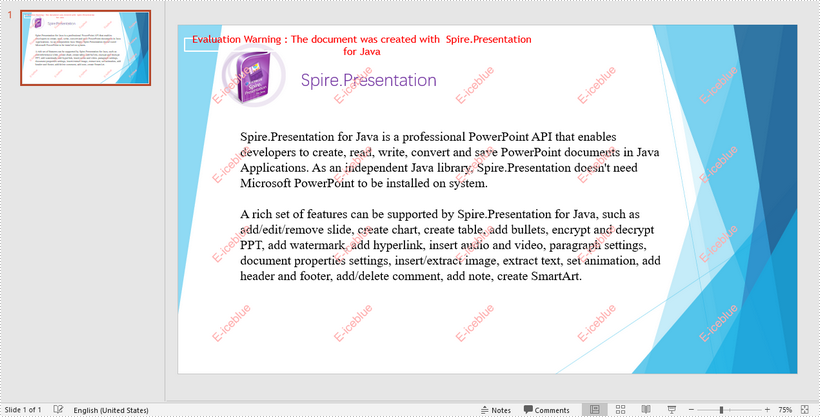Thursday, 06 January 2022 06:29

Java: Wrap or Unwrap Text in Excel Cells

In the process of manipulating Excel worksheets, sometimes you may encounter the situation where the text in a cell is so long that some of it is hidden. At this time, it’s recommended to wrap the extra-long text into multiple lines so you can see it all. This article will demonstrate how to programmatically wrap or unwrap text in Excel cells using Spire.XLS for Java.

Install Spire.XLS for Java

First of all, you're required to add the Spire.Xls.jar file as a dependency in your Java program. The JAR file can be downloaded from this link. If you use Maven, you can easily import the JAR file in your application by adding the following code to your project's pom.xml file.

<repositories>
<repository>
<id>com.e-iceblue</id>
<name>e-iceblue</name>
<url>https://repo.e-iceblue.com/nexus/content/groups/public/</url>
</repository>
</repositories>
<dependencies>
<dependency>
<groupId>e-iceblue</groupId>
<artifactId>spire.xls</artifactId>
<version>4.12.2</version>
</dependency>
</dependencies>

Wrap or Unwrap Text in Excel cells

Spire.XLS for Java supports wrapping or unwrapping text in Excel cells using the setWrapText() method provided by the IStyle interface. Below are detailed steps for your reference.

• Create a Workbook instance.
• Get a specific worksheet of the document using Workbook.getWorksheets().get() method.
• Get a specific cell of the worksheet using Worksheet.getRange().get() method.
• Get the style of the specified cell using XlsRange.getStyle() method and set whether the text is wrapped or not using setWrapText() method provided by IStyle interface.
• Save the document to another file using Workbook.saveToFile() method.
• Java
import com.spire.xls.ExcelVersion;
import com.spire.xls.Workbook;
import com.spire.xls.Worksheet;

public class WrapOrUnwrapText {
public static void main(String[] args) {
//Create a Workbook instance
Workbook workbook = new Workbook();

//Get the first worksheet
Worksheet sheet = workbook.getWorksheets().get(0);

//Wrap text in the cell "D8"
sheet.getRange().get("D8").getStyle().setWrapText(true);

//Unwrap text in the cell "D6"
sheet.getRange().get("D6").getStyle().setWrapText(false);

//Save the document to another file
workbook.saveToFile("output/WrapOrUnwrapText.xlsx", ExcelVersion.Version2013);
}
}